# 一、实验简介

ZYNQ系列嵌入式FPGA可以使PS将数据写入PL部分BRAM，PL可以将数据读取后再重新写入BRAM,PS将数据读出后再传走，这样可以使PL部分专心处理数据，不用考虑相对缓慢的数据接收与发送部分，并且可以实现数据的缓存。这也是数据量较小时的PS与PL的数据交互方法，当数据量达到BRAM的存储最大值后，就需要使用DDR来进行交互了。

# 二、vivado部分处理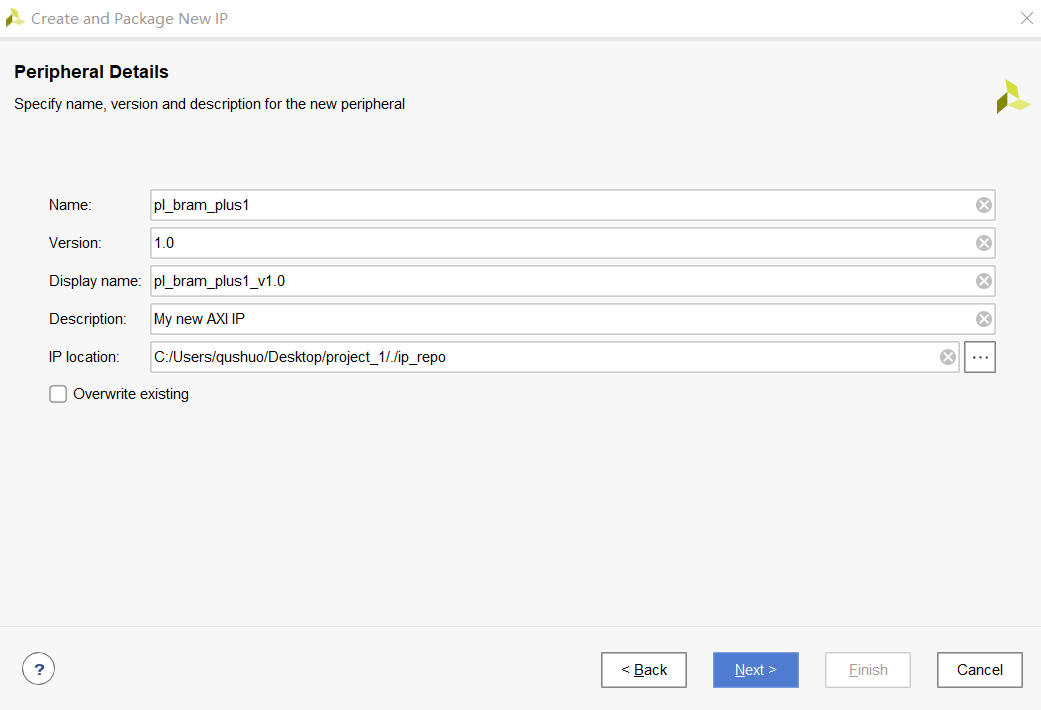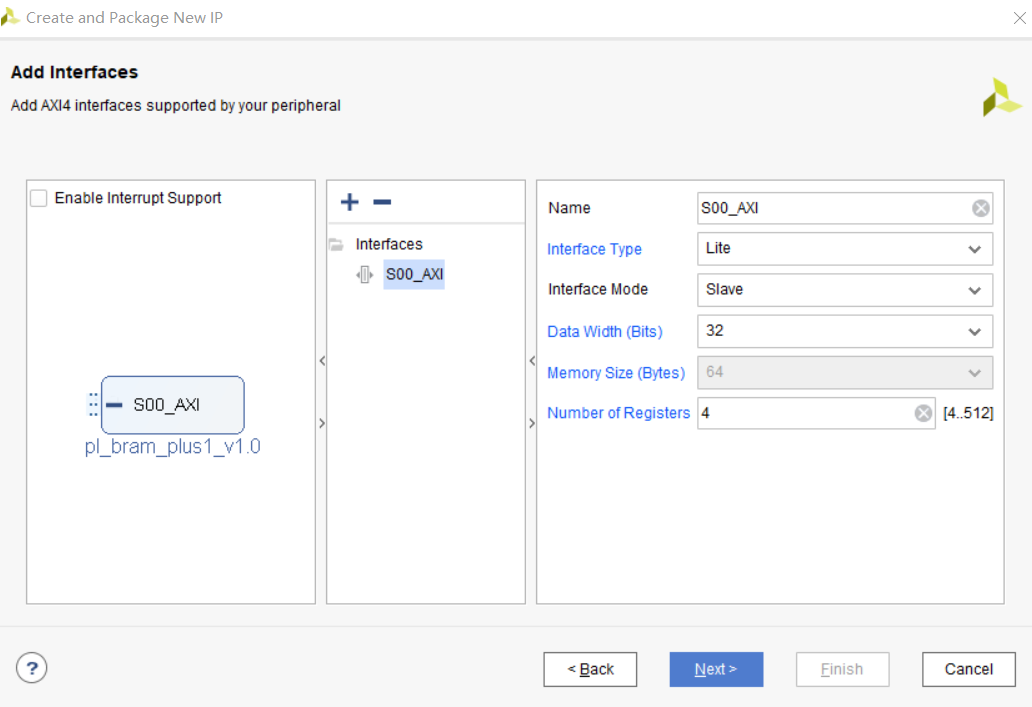output wire ram_clk ,                  //RAM 时钟
input wire [31:0] ram_rd_data,         //RAM 中读出的数据
output wire ram_en ,                   //RAM 使能信号
output wire [31:0] ram_addr ,          //RAM 地址
output wire [3:0] ram_we ,             //RAM 读写控制信号
output wire [31:0] ram_wr_data,        //RAM 写数据
output wire ram_rst ,                  //RAM 复位信号,高电平有

.ram_clk (ram_clk ),
.ram_rd_data (ram_rd_data),
.ram_en (ram_en ),
.ram_addr (ram_addr ),
.ram_we (ram_we ),
.ram_wr_data (ram_wr_data),
.ram_rst (ram_rst )

output wire ram_clk ,                //RAM 时钟
input wire [31:0] ram_rd_data,       //RAM 中读出的数据
output wire ram_en ,                 //RAM 使能信号
output wire [31:0] ram_addr ,        //RAM 地址
output wire [3:0] ram_we ,           //RAM 读写控制信号
output wire [31:0] ram_wr_data,      //RAM 写数据
output wire ram_rst ,                //RAM 复位信号,高电平有

在程序最后 Add user logic here 和 User logic ends 的中间行，添加如下代码：

bram_ip_plus1 inst_bram_ip_plus1(
.clk (S_AXI_ACLK),
.rst_n (S_AXI_ARESETN),
.start_rd (slv_reg0),
.start_addr (slv_reg1),
.rd_len (slv_reg2),
//RAM 端口
.ram_clk (ram_clk ),
.ram_rd_data (ram_rd_data),
.ram_en (ram_en ),
.ram_addr (ram_addr ),
.ram_we (ram_we ),
.ram_wr_data (ram_wr_data),
.ram_rst (ram_rst )
);

接下来在工程中创建一个新的模块，命名为“ bram_rd”，位于../BRAMIP/ip_repo/pl_bram_plus1_1.0/hdl路径下， bram_ip_plus1 模块的代码如下：

module bram_ip_plus1(
input clk , //时钟信号
input rst_n , //复位信号
input start_rd , //读开始信号
input [31:0] start_addr , //读起始地址
input [31:0] rd_len , //读数据的长度
//RAM 端口
output ram_clk , //RAM 时钟
input [31:0] ram_rd_data, //RAM 中读出的数据
output reg ram_en , //RAM 使能信号
output reg [31:0] ram_addr , //RAM 地址
output reg [3:0] ram_we , //RAM 读写控制信号
output reg [31:0] ram_wr_data, //RAM 写数据
output ram_rst //RAM 复位信号,高电平有效
);

//reg define
reg [1:0] flow_cnt;
reg start_rd_d0;
reg start_rd_d1;

//wire define
wire pos_start_rd;

//*****************************************************
//** main code
//*****************************************************

assign ram_rst = 1'b0;
assign ram_clk = clk ;
assign pos_start_rd = ~start_rd_d1 & start_rd_d0;

//延时两拍，采 start_rd 信号的上升沿
always @(posedge clk or negedge rst_n) begin
if(!rst_n) begin
start_rd_d0 <= 1'b0;
start_rd_d1 <= 1'b0;
end
else begin
start_rd_d0 <= start_rd;
start_rd_d1 <= start_rd_d0;
end
end

//根据读开始信号,从 RAM 中读出数据
always @(posedge clk or negedge rst_n) begin
if(!rst_n) begin
flow_cnt <= 2'd0;
ram_en <= 1'b0;
ram_addr <= 32'd0;
ram_we <= 4'd0;
end
else begin
case(flow_cnt)
2'd0 : begin
if(pos_start_rd) begin
ram_en <= 1'b1;
ram_addr <= start_addr;
flow_cnt <= flow_cnt + 2'd1;

end
end
2'd1 : begin
ram_wr_data <= ram_rd_data + 1;
flow_cnt <= flow_cnt + 2'd1;
ram_we <= 4'b1111;
end
2'd2:begin
if(ram_addr - start_addr == rd_len - 4) begin //数据读完
flow_cnt <= flow_cnt + 2'd1;
end
else begin
ram_addr <= ram_addr + 32'd4; //地址累加 4
ram_we <= 4'b0000;
flow_cnt <= flow_cnt - 2'd1;
end
end
2'd3 : begin
ram_addr <= 32'd0;
flow_cnt <= 2'd0;
ram_en <= 1'b0;
end
endcase
end
end

endmodule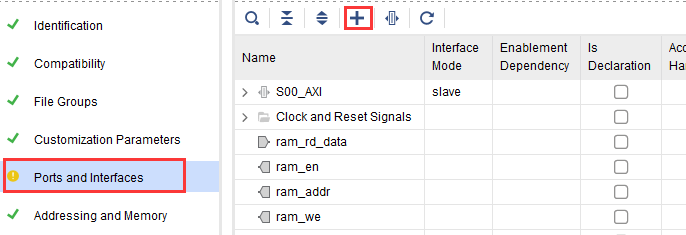在弹出的页面中，在 Name 一栏，输入 BRAM_PORT，然后点击 Interface Definition（总线定义）右侧的“ …”图标，如下图所示：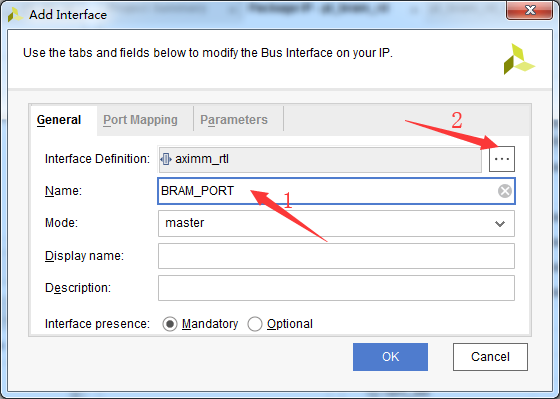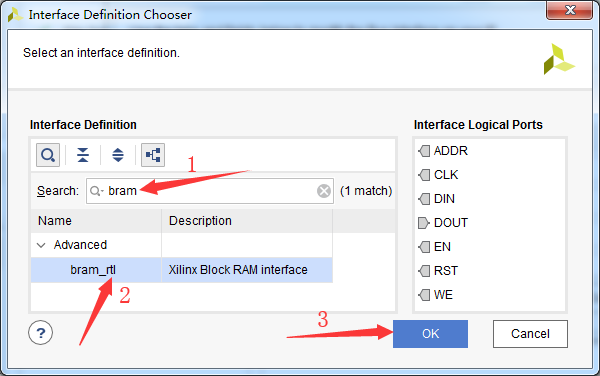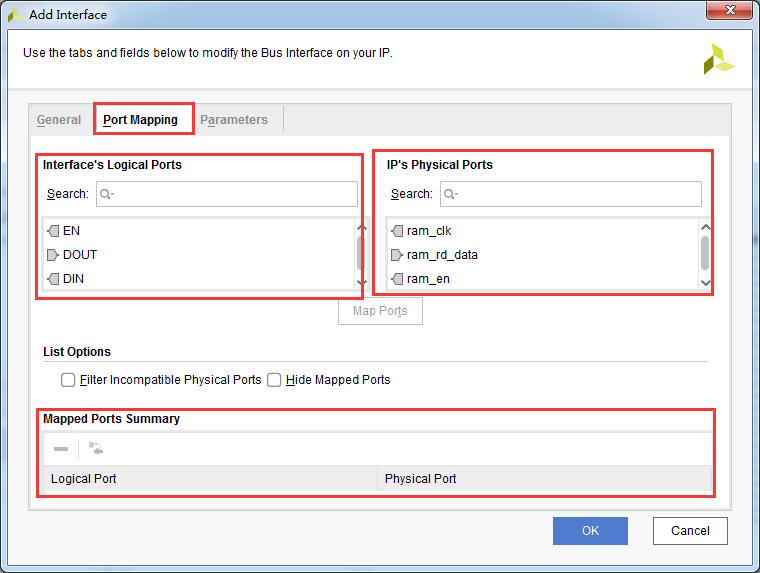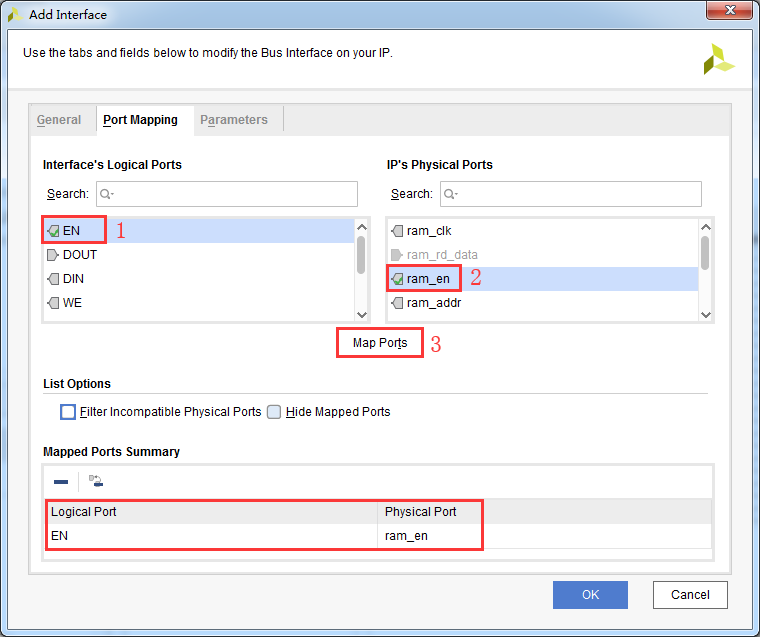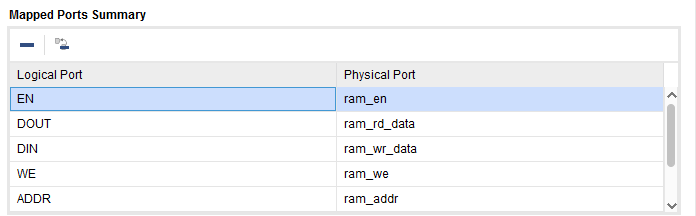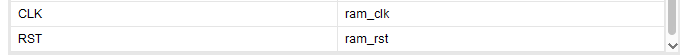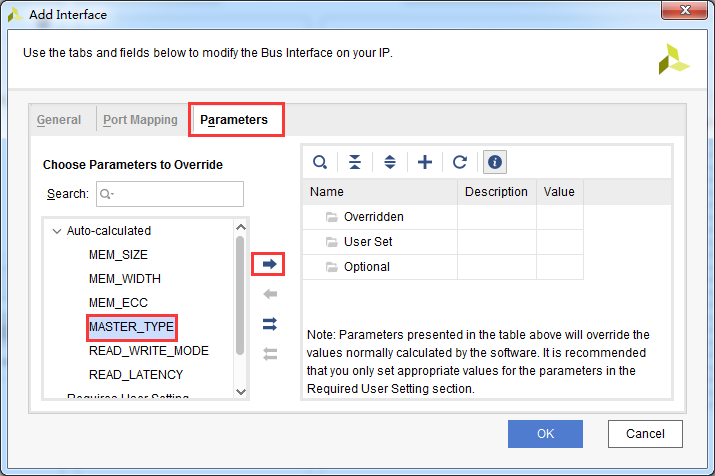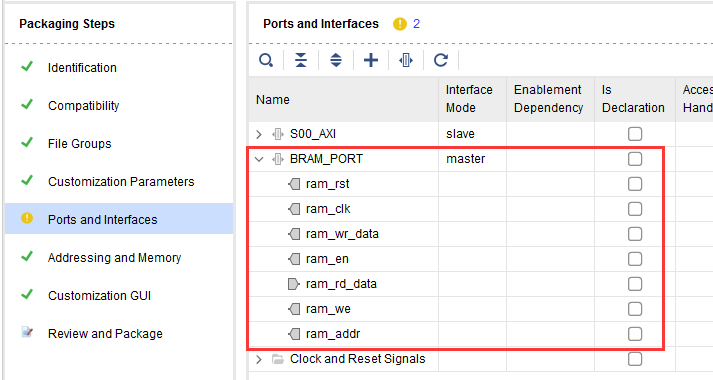接下来切换至“Review and Package”页面，点击上侧的“ IP has been modified”来更新 IP，最后点击“ Re-Package IP”完成 IP 核的封装，此时 IP 核的封装界面会自动关闭。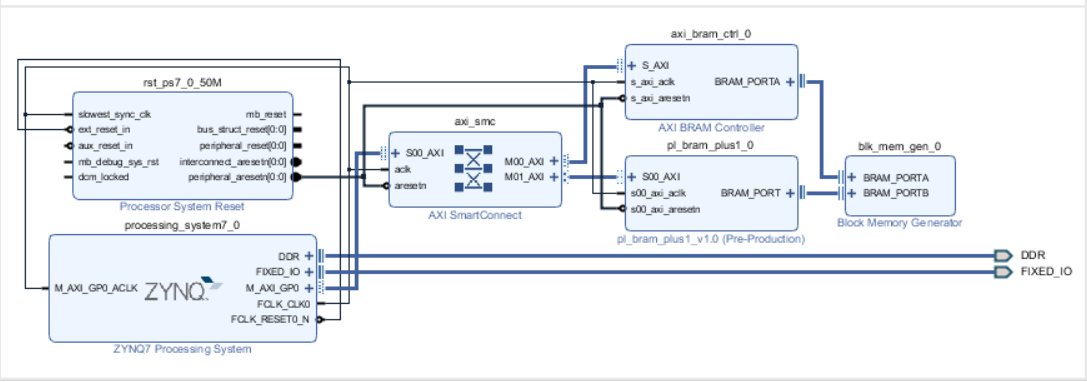# 三、SDK编程

SDK里先建立工程，我们建立一个空的或者HELLO WORLD!工程都可以，我选择建立空工程，然后在src里新建main.c，main.c的内容如下：

#include "xil_printf.h"
#include "stdio.h"
#include "pl_bram_plus1.h"
#include "xbram.h"
#include "xparameters.h"

#define PL_BRAM_BASE XPAR_PL_BRAM_PLUS1_0_S00_AXI_BASEADDR //PL_RAM_RD 基地址
#define PL_BRAM_START PL_BRAM_PLUS1_S00_AXI_SLV_REG0_OFFSET //RAM 读开始寄存器地址
#define PL_BRAM_START_ADDR PL_BRAM_PLUS1_S00_AXI_SLV_REG1_OFFSET //RAM 起始寄存器地址
#define PL_BRAM_LEN PL_BRAM_PLUS1_S00_AXI_SLV_REG2_OFFSET //PL 读 RAM 的深度

#define START_ADDR 0 //RAM 起始地址 范围:0~1023
#define BRAM_DATA_BYTE 4 //BRAM 数据字节个数

int data; //写入 BRAM 的字符数组
int data_len; //写入 BRAM 的字符个数

//main 函数
int main()
{
int i=0,wr_cnt = 0;
int read_data=0;
for(int k=0;k<1024;k++)
{
data[k] = k;
}
data_len = 1024;
//将用户输入的字符串写入 BRAM 中

//每次循环向 BRAM 中写入 1 个字符
for(i = BRAM_DATA_BYTE*START_ADDR ; i < BRAM_DATA_BYTE*(START_ADDR + data_len) ;i += BRAM_DATA_BYTE)
{
XBram_WriteReg(XPAR_BRAM_0_BASEADDR,i,data[wr_cnt]) ;
wr_cnt++;
}
//设置 BRAM 写入的字符串长度
PL_BRAM_PLUS1_mWriteReg(PL_BRAM_BASE, PL_BRAM_LEN , BRAM_DATA_BYTE*data_len) ;
//设置 BRAM 的起始地址
PL_BRAM_PLUS1_mWriteReg(PL_BRAM_BASE, PL_BRAM_START_ADDR, BRAM_DATA_BYTE*START_ADDR) ;
//拉高 BRAM 开始信号
PL_BRAM_PLUS1_mWriteReg(PL_BRAM_BASE, PL_BRAM_START , 1) ;
//拉低 BRAM 开始信号
PL_BRAM_PLUS1_mWriteReg(PL_BRAM_BASE, PL_BRAM_START , 0) ;
//从 BRAM 中读出数据
i = 0;
//循环从 BRAM 中读出数据
for(i = BRAM_DATA_BYTE*START_ADDR ; i < BRAM_DATA_BYTE*(START_ADDR + data_len) ;i += BRAM_DATA_BYTE)
{
read_data = XBram_ReadReg(XPAR_BRAM_0_BASEADDR,i) ;
printf("BRAM address is %d\t,Read data is %d\n",i/BRAM_DATA_BYTE ,read_data) ;
}
}


# 四、实验测试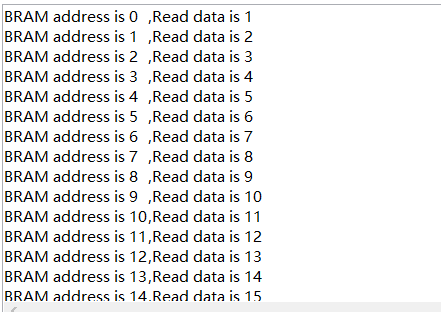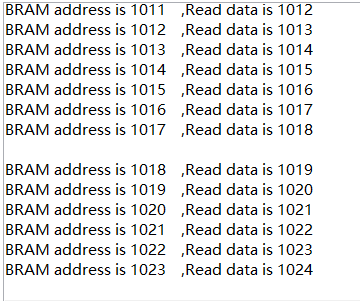# 五、总结09-142349
05-257514
05-211万+
12-202万+
09-16368
03-022303
01-241万+
08-30626
12-025638
©️2020 CSDN 皮肤主题: 书香水墨 设计师:CSDN官方博客